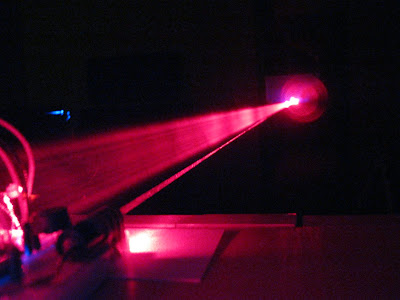## 2013-02-11

### Laser tripwire

Somebody call Catherine Zeta-Jones, because I have a laser tripwire.

I made it using an Arduino, a laser diode, a photocell (light dependent resistor) and a piezo buzzer. The laser and the detector are in the same place, so it requires a mirror to bounce the laser beam off (this way I only need power in one place). Here's what it looks like on a breadboard:The laser diode is just connected to 5V and ground, so it's always on. The piezo buzzer is connected to digital pin 3 on the Arduino and ground so that we can sound the alarm when the laser beam is broken. The photocell is connected in a voltage divider configuration. One leg of the photocell is connected to 5V, the other to ground through a resistor and to analog pin 0 on the Arduino. The way a photocell works is that it changes its resistance depending on light intensity. When the laser beam hits it, the resistance will be lower than when it doesn't. With the voltage divider, we can read a value on the Arduino's analog pin that will depend on the photocell's resistance and thus detect if the laser beam was broken.

The output voltage in the voltage divider depends on the values of the resistors in the following manner:

Vout = Rfixed/(Rphotocell+Rfixed)*Vin

Depending on the photocell's resistance range, we choose the value of the fixed resistor so that we can have a reasonable threshold value for the output voltage.

Here's the sketch that's running on the Arduino.
```const int PHOTOCELL_PIN = A0;
const int BUZZER_PIN = 3;
// voltage readings are in 0-1023 range
const int THRESHOLD = 500;

void setup() {
pinMode(PHOTOCELL_PIN, INPUT);
Serial.begin(9600);
}

long alarmEndTime = 0;

void loop() {
Serial.println(level);
long time = millis();
if (time < alarmEndTime) {
long timeLeft = alarmEndTime - time;
if (timeLeft % 1000 > 300) {
tone(BUZZER_PIN, 4000);
} else {
noTone(BUZZER_PIN);
}
} else {
noTone(BUZZER_PIN);
if (level < THRESHOLD) {
alarmEndTime = time + 3000;
}
}
}
```